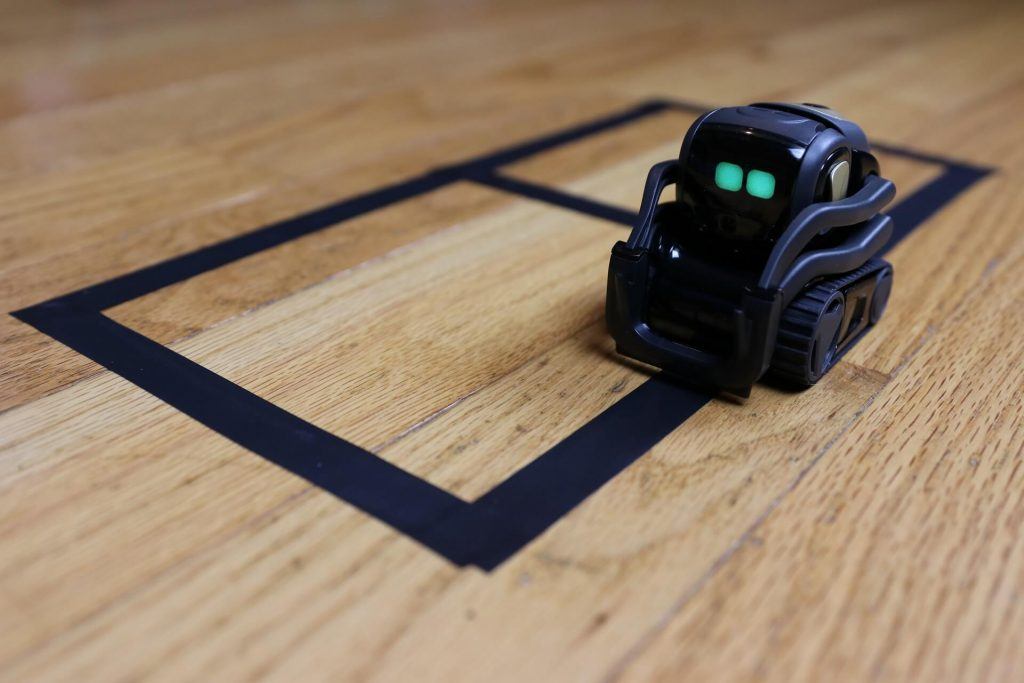# Anki Vector Drive Figure 8

Kinvert shows you how to use Anki Vector drive_straight() and turn_in_place() commands in the SDK to drive a figure 8. Come learn coding with Kinvert.

## Looking to Combing Drive_Straight() and Turn_In_Place()?

Learning robotics navigation is a fun and important skill. This is a nice fun nudge in that direction. What we’re doing here isn’t hard, yet there is a fair bit to learn in this example if you’re a beginner.

For this article, we will be using the Anki Vector SDK. This is one of many of Kinvert’s Anki Vector Examples in Python.

If you own a Cozmo but not a Vector, you should check out the Cozmo SDK.This is a quick and easy example. In addition, it helps students understand both for and lists in Python.

Let’s take a look at the commands a bit before diving in to the code.

What commands will we be using? Here they are:

• anki_vector.Robot.behavior.drive_straight()
• anki_vector.Robot.behavior.turn_in_place()
• for loops
• lists

We will link to the documentation once it is available online.

Keep in mind the argument we are passing is a value in radians per second.

We will use a combination of these commands to make Vector drive a figure 8 pattern.

## Drive_Straight() and Turn_In_Place() – Code

Let’s take a look at the code. We will be using anki_vector.Robot.behavior.drive_straight() and anki_vector.Robot.behavior.turn_in_place() to make Vector drive a figure 8. This one won’t be round, but sort of like a figure 8 like you’d see on an old digital alarm clock.

```"""
If you would like to use this code for
us for permission at:
www.kinvert.com/
"""

import anki_vector
from anki_vector.util import degrees, distance_mm, speed_mmps

def main():
args = anki_vector.util.parse_command_args()

with anki_vector.Robot(args.serial) as robot:
robot.behavior.drive_off_charger()

turns = (1,-1,-1,-1,-1,1,1,1) #This list says whether to turn left or right

for i in turns:
robot.behavior.drive_straight(distance_mm(50), speed_mmps(100))
robot.behavior.turn_in_place(degrees(i*90))

if __name__ == "__main__":
main()
```

What would you change to increase the size of the figure 8?

How would you get Vector to complete the figure 8 quicker?

How would you get Vector to start somewhere in the middle of the figure 8 instead of at an outer corner?

## Vectoring the Kinvert Way

At Kinvert we always like to spice things up and keep STEM fun. With all of our robots we’ve always done little victory dances after completing a goal. This is even more fun with the Anki Cozmo SDK and the Anki Vector SDK.

Think about how you could add a bit of fun after the exercise.

Here is what I did. The important thing to do is to make sure to add it after the for loop. Make sure to check your indentation or he could celebrate every time he turns. And come on, that’s obviously overdoing it.

animation = ‘anim_blackjack_victorwin_01’

robot.anim.play_animation(animation)

## In Conclusion

We hope you enjoyed this Anki Vector Example Using the SDK. We will be making many more in the near future.

If this example was helpful, or if you have any questions or custom modifications, please let us know in the comments below :).